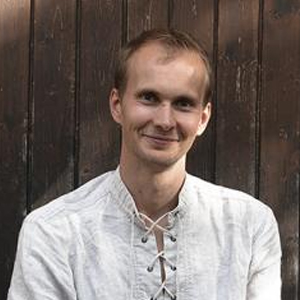# Juhan Aru

Probability theory, mathematical physics, random geometry. His research interests include how to mathematically describe and study systems where geometric structure and randomness interact. The mathematical field studying such phenomena lies at the interface of probability theory, analysis and mathematical physics. He works on random curves (SLE curves), height functions (Gaussian free field) and other random geometric structures (e.g. Gaussian multiplicative chaos), often with roots in the phenomena of statistical physics. Y6 Site Visit video: https://youtu.be/FSQazqcg9Nw